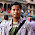## Markov Chains - Explained

Markov Chains is a probabilistic process, that relies on the current state to predict the next state. For Markov chains to be effective the current state has to be dependent on the previous state in some way; For instance, from experience we know that if it looks cloudy outside, the next state we expect is rain. We can also say that when the rain starts to subside into cloudiness, the next state will most likely be sunny. Not every process has the Markov Property, such as the Lottery, this weeks winning numbers have no dependence to the previous weeks winning numbers.

Usually when we have data, we calculate the probability of a state by counting the amount of times it occurs within a total of all states, we then end up with 0.5(50%) cloudy, 0.3(30%) rain, 0.2(20%) Sunny days of a year; Containing no information about when they occur relating to each other. To get the dependent probabilities, we count the number of times states occur relating to a state, and when we do that for all the states we then end up with a Markov Table :

To generate a simple prediction of events, we can say today is Cloudy, and from the table above we can determine that the next state will most likely be Rain with 50%, then we go to the Rain state, and the following day it will probably still be raining with 60%. Markov Chains can be represented as a state diagram, or a matrix called a Transition Matrix:

The Transition Matrix transitions from Row to Column as in the Markov Table. We can do calculations with a Transition Matrix utilizing a State Vector(vector of our current conditions) to give us the probabilities of the next states.

The above figure is set to 1(100%) cloudy for the current state, to calculate the probabilities of the next state we multiply the Current State Vector with the Matrix in figure 3:

State2 = Vector*Matrix
=[(1 *0.1 + 0*0.3 + 0*0.4); (1*0.5 + 0*0.6 + 0*0.1); (1*0.4 + 0*0.1 + 0*0.5)]
= [C;R;S] = [0.1; 0.5; 0.4]

No surprise, most likely rain. Now we can calculate the probabilities of the state after that using the resultant vector and multiplying it with the matrix in figure 3:

State3 =State2*Matrix
=[(0.1*0.1 + 0.5*0.3 + 0.4*0.4); (0.1*0.5 + 0.5*0.6 + 0.4*0.1); (0.1*0.4 + 0.5*0.1 + 0.4*0.5)]
= C;R;S = [0.32; 0.39; 0.29]

Rain most likely again, and we can calculate the probabilities of the state after that by multiplying with figure 2 again. That is the method of the Markov Chain of probabilities.

The Markov Chains that I have been working with are called 1st order Markov Chains, they only deal with 1 state to predict the next. In the above example, as you can see, when it transitions from cloudy to rain, it then absorbs into the rain state, never leaving leaving that state. The reason this happens is because the Transition Table only holds information of the last state, we don't know if it was sunny or raining before it was cloudy. you could have a 2nd order Markov Chain that would take the last two states and get the probability of the next states. All that is required is grouping the last two states into 1 state as in the example Table Below:

Markov Chains do go more in-depth and I've only touched the surface. When programming Markov Chains most developers use the table method, linking a list of states to its list of next state probabilities. One toy program that people like to mention synonymous with Markov Chains is the Markov Chain text generator, trained on text, basically the states are words, and each word is linked to a list of words that have appeared after it in the training text.

Here`s a quote from my 3rd Order Markov Chain Text Generator trained on the Bible "Then Joshua said to the olive-tree, Be king over us."

This tutorial is not complete - I will be adding pseudo-code, and turning the calculations into images.

http://en.wikipedia.org/wiki/Markov_chain

1.Just keep going. All posts are simple to understand.

2.Would love to see the code to generate that quote.

3.Great article!

4.5.Thank you for the effort. Your blog looks very promissing.

6.Excellent work explaining Markov chains. This is the first time I understand a concept online in one read through.

7.Lucid explaination. Thank you.

8.really this illustration is simple and benefit .... i need such a same tutorial about hidden markov model. it is related to my thesis and i find a difficulty to understand it .. or you can saggest me any other one .... thanks

9.Best introduction in Markov chains so far. Thank you!

10.Keep writing more....
Thank you.﻿

11.Thank you for writing this. It is probably the most illuminating introduction that I could find.

12.Well explained with really brain friendly way. Thank you.

13.Great post- thanks for sharing!

14.Your blog is amazing. It is really worth reading. Hope you would like to read Markov Chains

15.You are genius thanks dude..!

16.Thanks a lot, very useful !

17.nice 4 beginners

18.I think you understand

ป๊อกเด้ง
Royal1688

Thank you very much

19.ปรับรูปหน้าเรียว
ฉีดหน้าเรียว ลดริ้วรอย เป็น

ต้องการ

หน้าเรียวทำเป็น

ยังไง

หลายคน

อาจจะ

กำเนิด

ปัญหา

นี้
และก็

การฉีดลดหน้าเรียวด้วยโบท็อกก็เป็นขั้นตอนการ

รักษาที่ทำเป็น

ง่าย โดยไม่ต้องผ่าตัด
สารารถยนต์

ช่วยลดลางเลือน

ริ้วรอยรวมทั้ง

ร่องลึกได้ภายในช่วงระยะเวลา

เร็วทันใจ

ได้ผล

การดูแลรักษา

ที่น่าพอใจ

ละสร้างความเชื่อมั่น

ให้กับผู้มีปัญหาได้อย่างดีเยี่ยม

สารที่ฉีดลดหน้าเรียว
ยังเป็นสารจากธรรมชาติที่เป็นโปรตีนบริสุทธิ์สกัดจากแบคทีเรียที่มีคุณประโยชน์

ประเภทหนึ่ง

ซึ่งจะช่วยเหมือน

กล้าม

โบท็อก กังนัม
โบท็อค pantip
โบท็อค ลดกราม